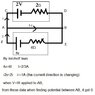# Potential difference

• kalupahana

## Homework Statement

The Cells in a circuit has 2V and 4V emfs and 2Ω and 6Ω internal resistance respectively. Find the potential difference between AB

V=IR
Kirchoff's Laws

## The Attempt at a Solution

The attempts are in image

#### Attachments

•Untitled.jpg
20.2 KB · Views: 368

AB seems to be a shortcircuit. That is a potential difference of 0, so if you end up getting that, it's nothing to worry about. If you got something other than 0, that's when you should be worried.Basically, you can think of a shortcircuit as taking a branch with a single resistor R, and doing R → 0. From V = IR, you see that I = V/R, so if R → 0, the only way to stop I from going to infinity and being a defined finite value, is to have V = 0, that is, the potential difference across a shortcircuit vanishes.

AB seems to be a shortcircuit. That is a potential difference of 0, so if you end up getting that, it's nothing to worry about. If you got something other than 0, that's when you should be worried.Basically, you can think of a shortcircuit as taking a branch with a single resistor R, and doing R → 0. From V = IR, you see that I = V/R, so if R → 0, the only way to stop I from going to infinity and being a defined finite value, is to have V = 0, that is, the potential difference across a shortcircuit vanishes.
If VAB ≠ 0, then it would be I-i that goes to infinity.

The I in my explanation was the I of an arbitrary branch in which a shortcircuit is produced, I didn't use the same circuit as the one given. That's why I said V = IR, and not V = (I-i)R.

The I of my example would be extrapolated to whatever it need be, per particular circuit.

Ok thanks for help, i got it

You're welcome.